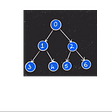# Algorithm_Leetcode

1207. Unique Number of Occurrences

Given an array of integers `arr`, write a function that returns `true` if and only if the number of occurrences of each value in the array is unique.

Example 1:

`Input: arr = [1,2,2,1,1,3]Output: trueExplanation: The value 1 has 3 occurrences, 2 has 2 and 3 has 1. No two values have the same number of occurrences.`

Example 2:

`Input: arr = [1,2]Output: false`

Example 3:

`Input: arr = [-3,0,1,-3,1,1,1,-3,10,0]Output: true`

Constraints:

• `1 <= arr.length <= 1000`
• `-1000 <= arr[i] <= 1000`

1403. Minimum Subsequence in Non-Increasing Order

Given the array `nums`, obtain a subsequence of the array whose sum of elements is strictly greater than the sum of the non included elements in such subsequence.

If there are multiple solutions, return the subsequence with minimum size and if there still exist multiple solutions, return the subsequence with the maximum total sum of all its elements. A subsequence of an array can be obtained by erasing some (possibly zero) elements from the array.

Note that the solution with the given constraints is guaranteed to be unique. Also return the answer sorted in non-increasing order.

Example 1:

`Input: nums = [4,3,10,9,8]Output: [10,9] Explanation: The subsequences [10,9] and [10,8] are minimal such that the sum of their elements is strictly greater than the sum of elements not included, however, the subsequence [10,9] has the maximum total sum of its elements.`

Example 2:

`Input: nums = [4,4,7,6,7]Output: [7,7,6] Explanation: The subsequence [7,7] has the sum of its elements equal to 14 which is not strictly greater than the sum of elements not included (14 = 4 + 4 + 6). Therefore, the subsequence [7,6,7] is the minimal satisfying the conditions. Note the subsequence has to returned in non-decreasing order.`

Example 3:

`Input: nums = Output: `

Constraints:

• `1 <= nums.length <= 500`
• `1 <= nums[i] <= 100`

98. Validate Binary Search Tree

Given the `root` of a binary tree, determine if it is a valid binary search tree (BST).

A valid BST is defined as follows:

• The left subtree of a node contains only nodes with keys less than the node’s key.
• The right subtree of a node contains only nodes with keys greater than the node’s key.
• Both the left and right subtrees must also be binary search trees.

Example 1:

`Input: root = [2,1,3]Output: true`

Example 2:

`Input: root = [5,1,4,null,null,3,6]Output: falseExplanation: The root node's value is 5 but its right child's value is 4.`

Constraints:

• The number of nodes in the tree is in the range `[1, 104]`.
• `-231 <= Node.val <= 231 - 1`

--

--

--

## More from Joo Hee Paige Kim

Consistency achieves everything https://github.com/paigekim29

Love podcasts or audiobooks? Learn on the go with our new app.

## Beginner’s Guide For Software Testing## Deploy Kubernetes with Ansible## Resources for Google Summer of Code## Git — The forefront of version control systems## Scheduling things in a Serverless environment/Lambdas## How to Begin Editing a Document in Python## Generate a EAN-13 code barcode as PNG file in Python## How to Retrieve the Sitemap URL for an HTML Document in C# .NET Framework## Joo Hee Paige Kim

Consistency achieves everything https://github.com/paigekim29

## The story of time complexity## Interval Scheduling Greedy Algorithm